Electron. J. Diff. Equ., Vol. 2014 (2014), No. 206, pp. 1-14.

### Precise asymptotic behavior of strongly decreasing solutions of first-order nonlinear functional differential equations George E. Chatzarakis, Kusano Takasi, Ioannis P. Stavroulakis

Abstract:
In this article, we study the asymptotic behavior of strongly decreasing solutions of the first-order nonlinear functional differential equation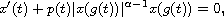whereis a positive constant such that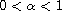, p(t) is a positive continuous function on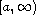, a>0 and g(t) is a positive continuous function onsuch that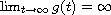. Conditions which guarantee the existence of strongly decreasing solutions are established, and theorems are stated on the asymptotic behavior of such solutions, at infinity. The problem it is studied in the framework of regular variation, assuming that the coefficient p(t) is a regularly varying function, and focusing on strongly decreasing solutions that are regularly varying. In addition, g(t) is required to satisfy the condition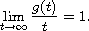Examples illustrating the results are also given.

Submitted June 26, 2014. Published October 2, 2014.
Math Subject Classifications: 34C11, 26A12
Key Words: Functional differential equation; strongly decreasing solution; regularly varying function; slowly varying solution.

Show me the PDF file (272 KB), TEX file, and other files for this article.George E. Chatzarakis Department of Electrical and Electronic Engineering Educators School of Pedagogical and Technological Education (ASPETE) 14121, N. Heraklio, Athens, Greece email: geaxatz@otenet.gr, gea.xatz@aspete.gr Kusano Takasi Professor Emeritus at: Department of Mathematics Faculty of Science, Hiroshima University Higashi-Hiroshima 739-8526, Japan email: kusanot@zj8.so-net.ne.jp Ioannis P. Stavroulakis Department of Mathematics, University of Ioannina 451 10 Ioannina, Greece email: ipstav@cc.uoi.gr